# How to Plot a Histogram in Python

Plotting a histogram in python is very easy. I will talk about two libraries - matplotlib and seaborn. Plotting is very easy using these two libraries once we have the data in the Python pandas dataframe format.

I will be using college.csv data which has details about university admissions.

In :
import pandas as pd

In :
df = pd.read_csv('College.csv')

In :
df.head(1)

Out:
Unnamed: 0 Private Apps Accept Enroll Top10perc Top25perc F.Undergrad P.Undergrad Outstate Room.Board Books Personal PhD Terminal S.F.Ratio perc.alumni Expend Grad.Rate
0 Abilene Christian University Yes 1660 1232 721 23 52 2885 537 7440 3300 450 2200 70 78 18.1 12 7041 60

Ok we have the data in the dataframe format. Lets start with our histogram tutorial.

## How to plot histogram in Python using Matplotlib

Lets first import the library matplotlib.pyplot.

Note:You don't need %matplotlib inline in Python3+ to display plots in jupyter notebook.

In :
import matplotlib.pyplot as plt


Lets just pick one column from dataframe and plot using matplotlib. We will use plot() method which can be used both on Pandas Dataframe and Series. In the below example, we are applying plot() on Pandas Series data type.

There are two ways to use plot() method. Either directly on the dataframe or pass dataframe to plt.plot() function.

Lets first try the dataframe.plot() method.

In :
df['Apps'].plot(kind='hist')

Out:
<matplotlib.axes._subplots.AxesSubplot at 0x7f3b2ee661d0>df.plot() has many options. Do df.plot? to find the help and its usage.

One important parameter when plotting a histogram is number of bins. By default plot() divides the data in 10 bins.

We can control this parameter using bins parameter. Lets try bins=5

In :
df['Apps'].plot(kind='hist',bins=5)

Out:
<matplotlib.axes._subplots.AxesSubplot at 0x7f3b2f3772d0>Note the difference we see only two bars and bars look bigger, if we increase the plot() number of bins, we would see more number of smaller bars becasue the data will be divided in two more number of bins. We can see data more granularally.

In :
df['Apps'].plot(kind='hist',bins=15)

Out:
<matplotlib.axes._subplots.AxesSubplot at 0x7f3b2f560a90>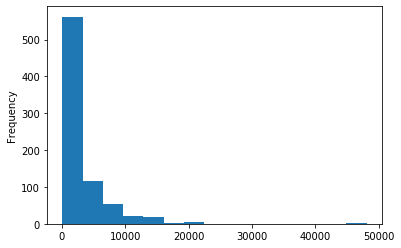Ok thats that. Lets try plt.plot() method. This gives us more flexibility and more options to control the plot figure. Lets start simple and use plt.plot() method to draw the histogram of the same column.

In :
plt.plot(df['Apps'])

Out:
[<matplotlib.lines.Line2D at 0x7f3b2e169310>]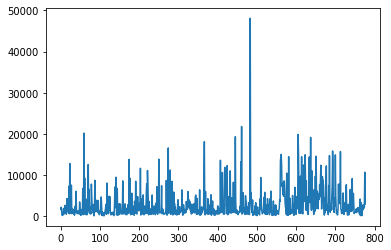Oops, we got the line plot. For histogram plotting, there is hist() method of pyplot. Lets try that.

In :
plt.hist(df['Apps'])

Out:
(array([638.,  92.,  31.,  11.,   4.,   0.,   0.,   0.,   0.,   1.]),
array([   81. ,  4882.3,  9683.6, 14484.9, 19286.2, 24087.5, 28888.8,
33690.1, 38491.4, 43292.7, 48094. ]),
<a list of 10 Patch objects>)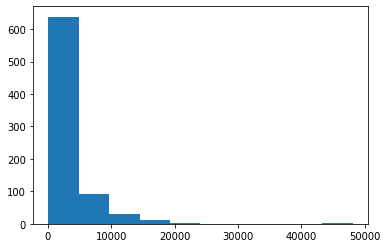Ok we got our histogram back. We can pass in the bins parameter to pyplot to control the bins.

In :
plt.hist(df['Apps'],bins=5)

Out:
(array([730.,  42.,   4.,   0.,   1.]),
array([   81. ,  9683.6, 19286.2, 28888.8, 38491.4, 48094. ]),
<a list of 5 Patch objects>)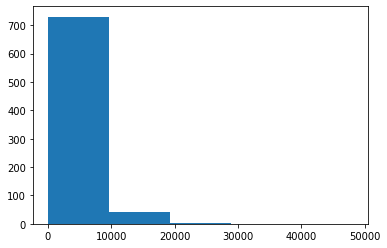Matplotlib is a great package to control both axes and figure of the plot. By the way, figure is the bounding box and axes are the two axes, shown in the plot above. Matplotlib gives access to both of these objects. For example we can control the matplotlib figure size using figsize options.

In :
fig, ax = plt.subplots(figsize=(5,3))
plt.hist(df['Apps'],bins=5)

Out:
(array([730.,  42.,   4.,   0.,   1.]),
array([   81. ,  9683.6, 19286.2, 28888.8, 38491.4, 48094. ]),
<a list of 5 Patch objects>)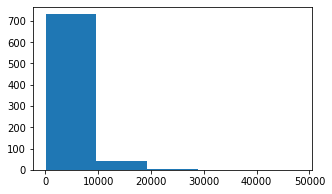As you noted above the size of the plot has been reduced. There is much that we can do with fig,ax objects. I will have to write a complete series on it to touch upon those options. Lets just for now move on to 2nd way of plotting the python plots.

## How to plot histogram in Python using Seaborn

Matplotlib where gives us lot of control, Searborn is quick and easy to draw beautiful plots right out of the box.

Lets just import the library first.

In :
import seaborn as sns

In [ ]:
Searborn has named it distplot instead of hist plot. displot stands for distribution plot.

In :
sns.distplot(df['Apps'])

Out:
<matplotlib.axes._subplots.AxesSubplot at 0x7f3b287e5250>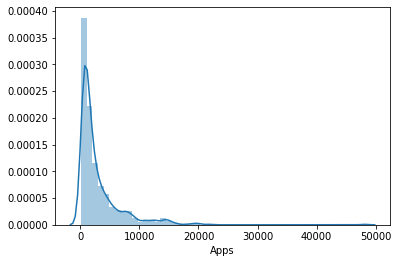If you see above, the seaborn distribution plot looks quit different from the matplotlib histogram plot. The line over the histogram is called density line. Lets just remove the line with option kde=False.

In :
sns.distplot(df['Apps'],kde=False)

Out:
<matplotlib.axes._subplots.AxesSubplot at 0x7f3b2acb24d0>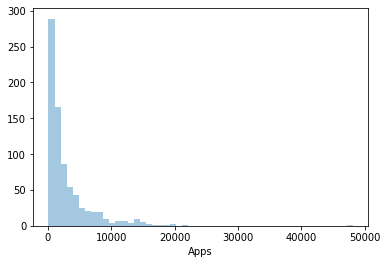The y axis also looks better in seaborn plot. With kde=True, seaborn was showing density on the yaxis as opposed to frequency.

As usual, we can control the bins with bins option in seaborn. Lets try bins=5.

In :
sns.distplot(df['Apps'],kde=False,bins=5)

Out:
<matplotlib.axes._subplots.AxesSubplot at 0x7f3b2ac52d10>Remember seaborn uses matplotlib objects under the hood. Therefore we can still control the plot using pyplot object.

In :
sns.distplot(df['Apps'],kde=False,bins=5)
plt.xlabel('No of Univ Applications')

Out:
Text(0.5, 0, 'No of Univ Applications')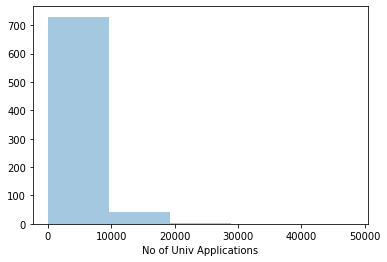As we see above, we changed the x-axis label by using the xlabel method of plt.

## Wrap Up!

In the above tutorial, I have shown you how to plot histograms in Python using two libraries Matplotlib and Seaborn . Hope you would find it useful.